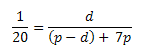# Mixing Ratio and Concentration Calculator

Skip ahead to the Pressure Washer Dilution Calculator.

This seemingly simple subject is still the cause of confusion, and it was for me too. After having to figure this out two springs in a row I decided to write it down and share it. Consider this problem: One part pressure washer detergent should be mixed with 20 parts water for proper dilution (1:20). The pressure washer uses a fixed mixing ratio of 1:7 detergent to water. How should the detergent be pre-diluted so that the pressure washer delivers a spray with 1:20 mixing ratio?

It is easy to think that it is a simple algebra problem, using the equation 1/20 = (1/7) × MR. Not so! This would have worked had we used concentration instead of mixing ratio, however.

### Definitions:

• Mixing Ratio, MR = solute / solvent
• Concentration, C = solute / (solvent + solute)

Mixing ratio is usually given as the inverse, solvent/solute, but either one works as long as we know which one we're using.
Concentration is often expressed in percent by multiplying C by 100%.

## Pressure Washer Dilution

### Theory, Using Mixing Ratio

The way to think about the problem in the introduction is to think about the total quantities of detergent and water used. Let's say we want to prepare a p gallon jug of pre-mix detergent and water.On the left hand side we have the desired final mixing ratio. On the right hand side we have the quantities of detergent and water in units of gallon, where d is the quantity of detergent in the p gallon jug. The (p-d) is the quantity of water in the jug, and 7p is the number of gallons added by the pressure washer while dispensing the content of the jug. If we set p=1 gallon, we solve for d and find that d=0.381 gal = 48.8 oz.

### Theory, Using Concentration

We convert the mixing ratios to concentrations and we have......where C is the concentration of detergent in the pre-mix jug, C = d/p.

Solving for C we find that C=0.381 (or 38.1%). Multiply 1 gallon by 0.381 and we find that we need 0.381 gal detergent = 48.8 oz.

This type of problem is easier to solve using concentration rather than mixing ratios, but since directions provided with consumer products use mixing ratios (in the U.S. anyway) we will use them as well.

## Pressure Washer Dilution Calculator

This calculator computes the amount of detergent needed in the pre-mix jug, using the given mixing ratios.

Detergent Water
Final Mixing Ratio: :
Pressure Washer Mixing Ratio: :
Tip: Set to 1:0 for a general mixing calculation.
Desired Volume of Pre-mix:  gal

 Pre-mix Concentration: % Detergent: gal†= fl. oz Water: gal= fl. oz

The water volume needs typically not be measured since we can just top up the jug with water.

The calculator also works for mixing gasoline and oil for two-stroke engines, for example. Set the pressure washer mixing ratio to 1:0. Notice that this calculator works differently than the typical gasoline pre-mix calculator on the web in that you set the final volume of pre-mix rather than the volume of gasoline (or water) used.

The unit here is intended to be gallon (128 fl. oz,) but it can be any unit including liter. Naturally, the fl. oz result only applies if the unit is gallon.